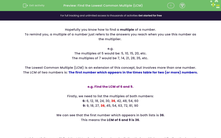# Find the Lowest Common Multiple (LCM)

In this worksheet, students will find the LCMs of pairs of numbers by listing their multiples and finding the lowest multiple which appears in both.Key stage:  KS 4

Year:  GCSE

GCSE Subjects:   Maths

GCSE Boards:   Pearson Edexcel, OCR, Eduqas, AQA,

Curriculum topic:   Number, Number Operations and Integers

Curriculum subtopic:   Structure and Calculation Whole Number Theory

Difficulty level:#### Worksheet Overview

Hopefully you know how to find a multiple of a number.

To remind you, a multiple of a number just refers to the answers you reach when you use this number as the multiplier.

e.g.

The multiples of 5 would be: 5, 10, 15, 20, etc.

The multiples of 7 would be 7, 14, 21, 28, 35, etc.

The Lowest Common Multiple (LCM) is an extension of this concept, but involves more than one number.

The LCM of two numbers is: The first number which appears in the times table for two (or more) numbers.

e.g. Find the LCM of 6 and 9.

Firstly, we need to list the multiples of both numbers:

6: 6, 12, 18, 24, 30, 36, 42, 48, 54, 60

9: 9, 18, 27, 36, 45, 54, 63, 72, 81, 90

We can see that the first number which appears in both lists is 36.

This means the LCM of 6 and 9 is 36.

In this activity, you will find the LCMs of pairs of numbers by listing their multiples and finding the lowest multiple which appears in both.

You may want to have a pen and paper handy so that you can write out your lists to help you answer these questions.

### What is EdPlace?

We're your National Curriculum aligned online education content provider helping each child succeed in English, maths and science from year 1 to GCSE. With an EdPlace account you’ll be able to track and measure progress, helping each child achieve their best. We build confidence and attainment by personalising each child’s learning at a level that suits them.

Get started